# NCERT Exemplar Class 12 Physics Solutions for Chapter 7 Alternating Current

The NCERT Class 12 Physics Exemplar for Chapter 7 Alternating Current is compiled by the subject experts at BYJU’S. These exemplar problems have questions from the NCERT Exemplar Class 12 Physics book, together with important questions from previous years’ question papers and sample papers.

Students need to study these exemplar questions , as many times, similar questions are asked both in the Class 12 board examination as well as national-level graduate entrance examinations. This solution has different forms of questions like MCQs, fill in the blanks, match the following, numerical problems and exercises.

## Download the PDF of the NCERT Exemplar for Class 12 Physics Chapter 7 Alternating Current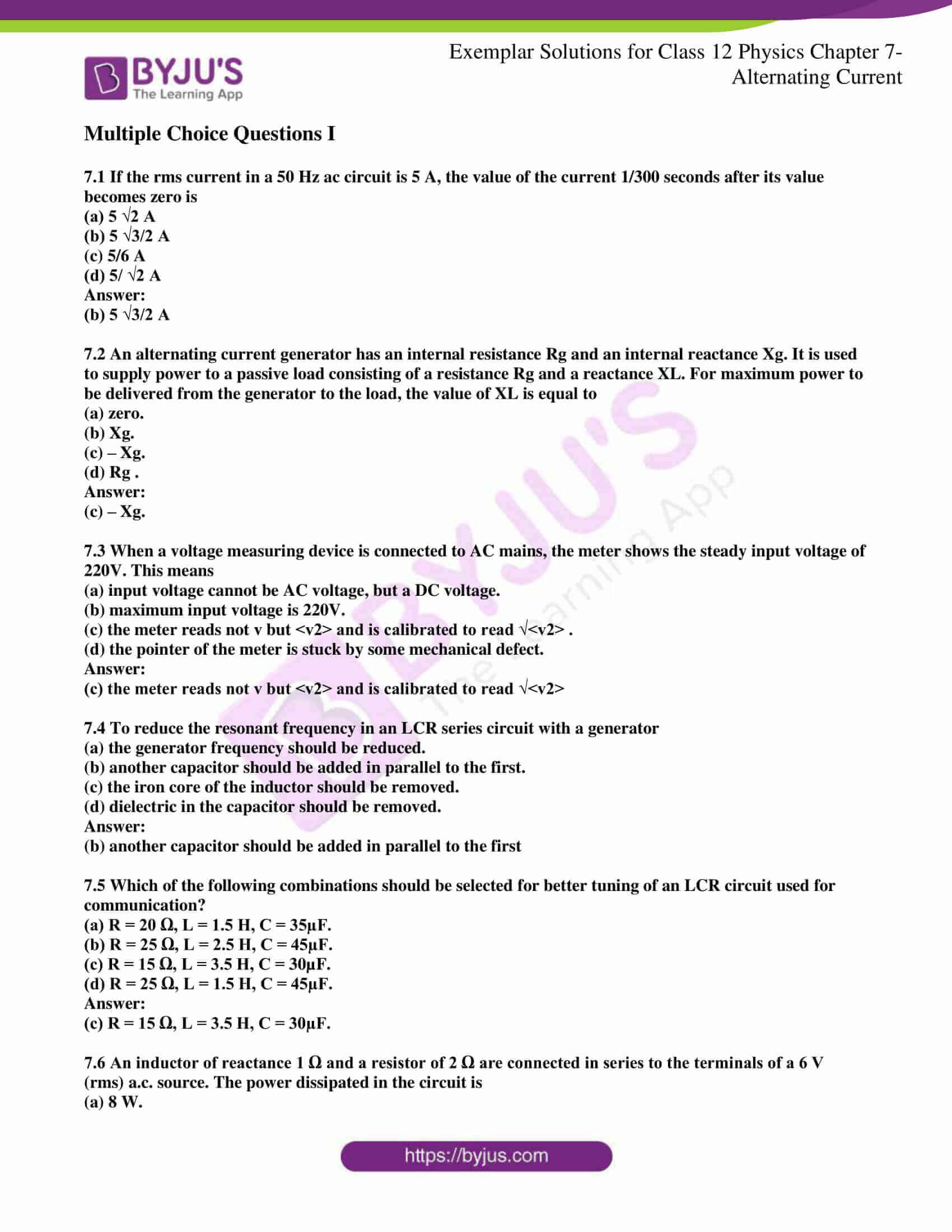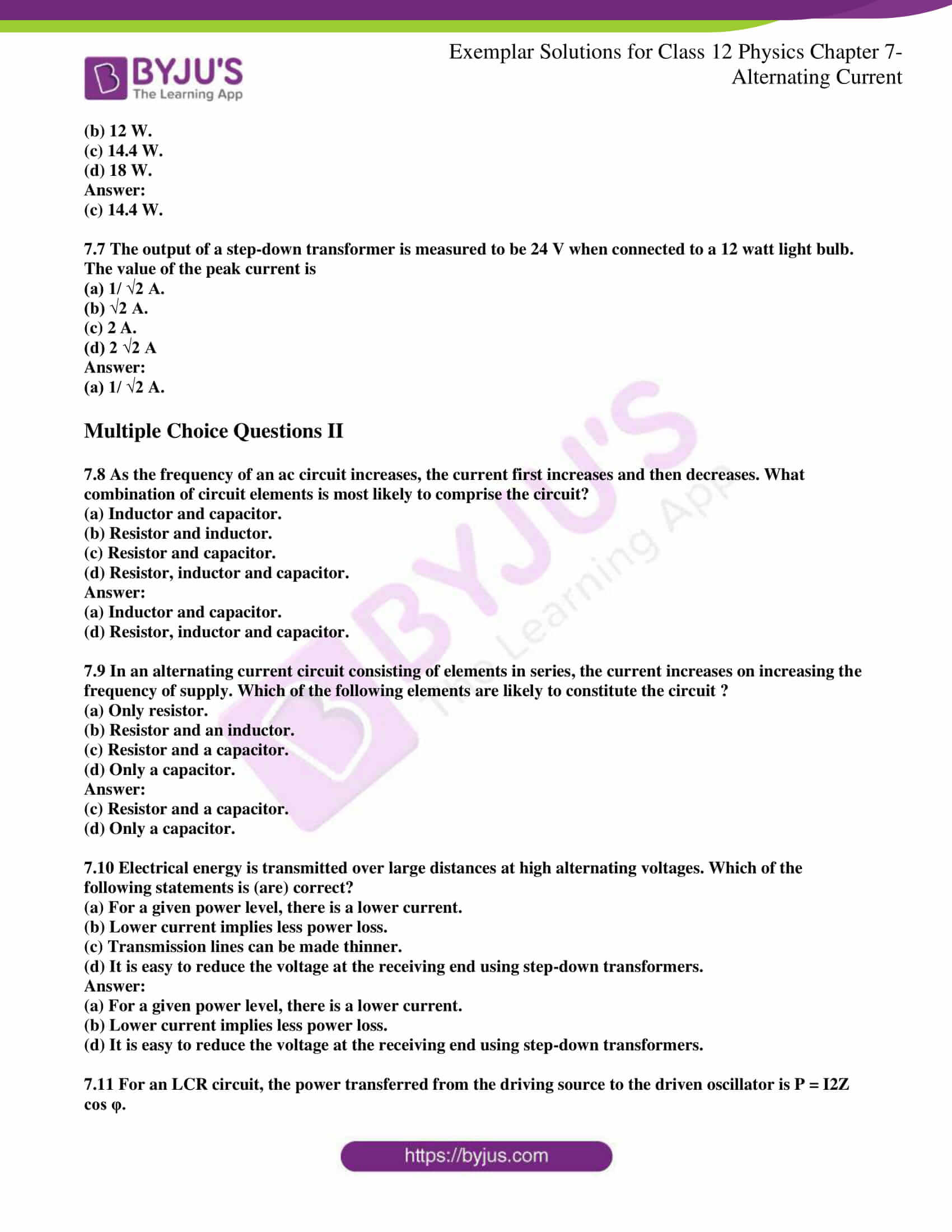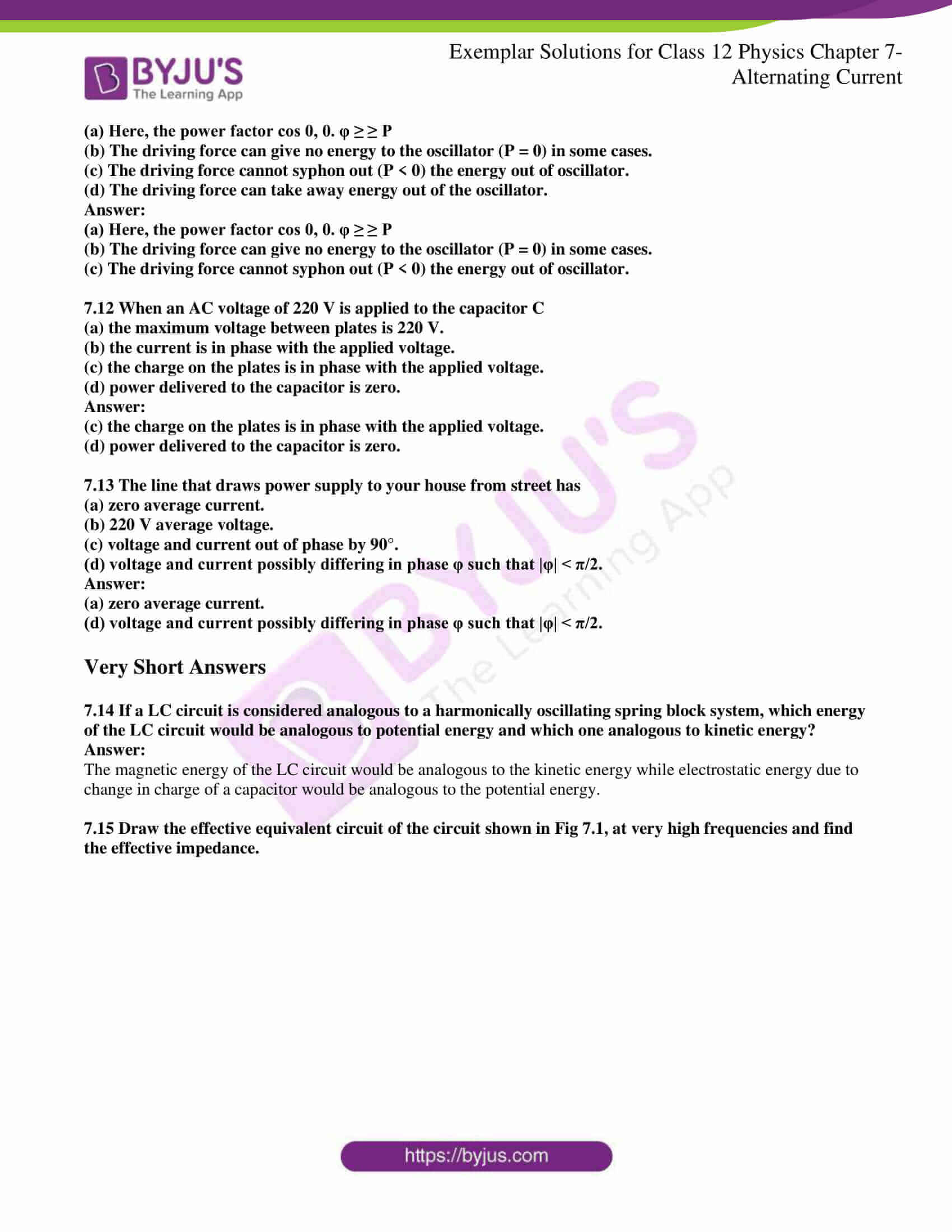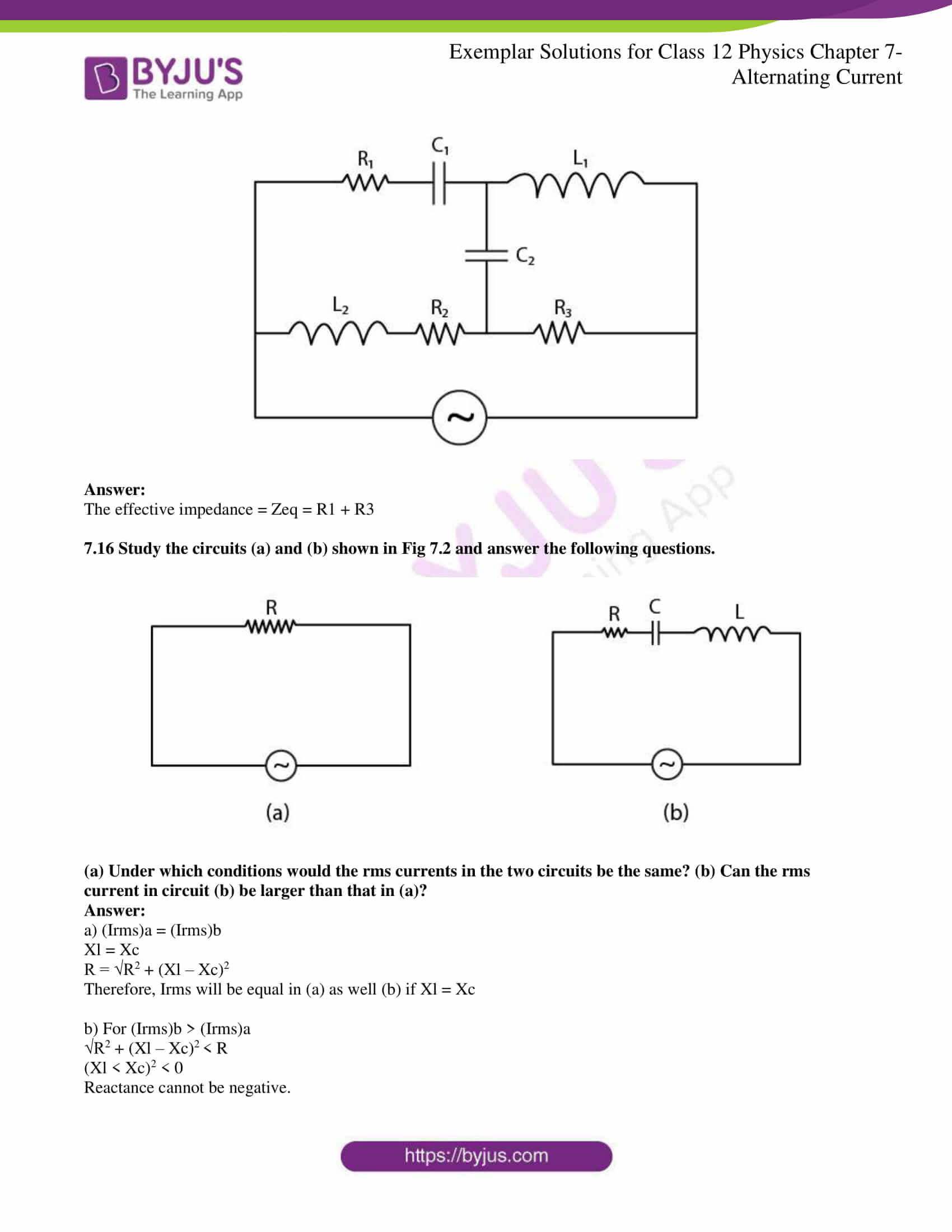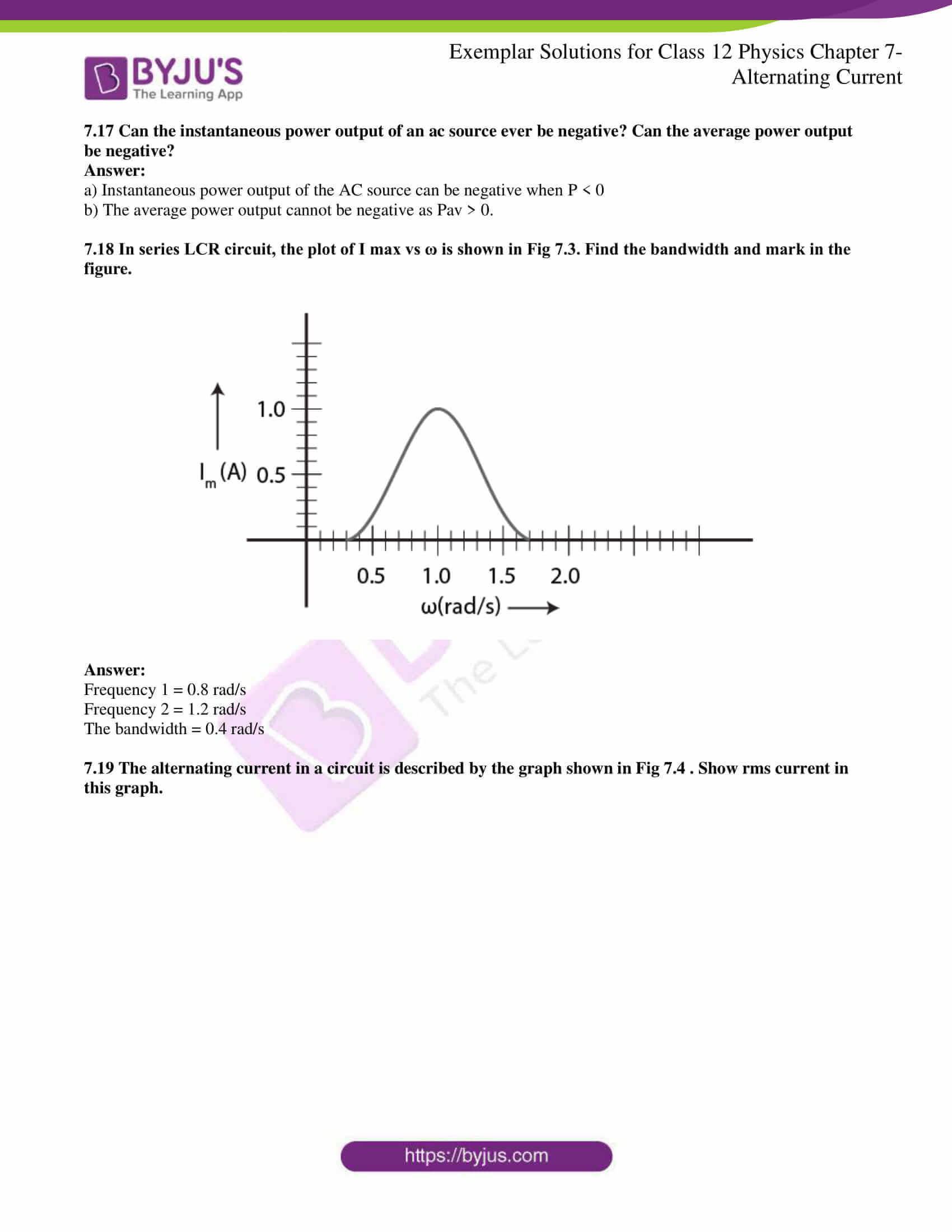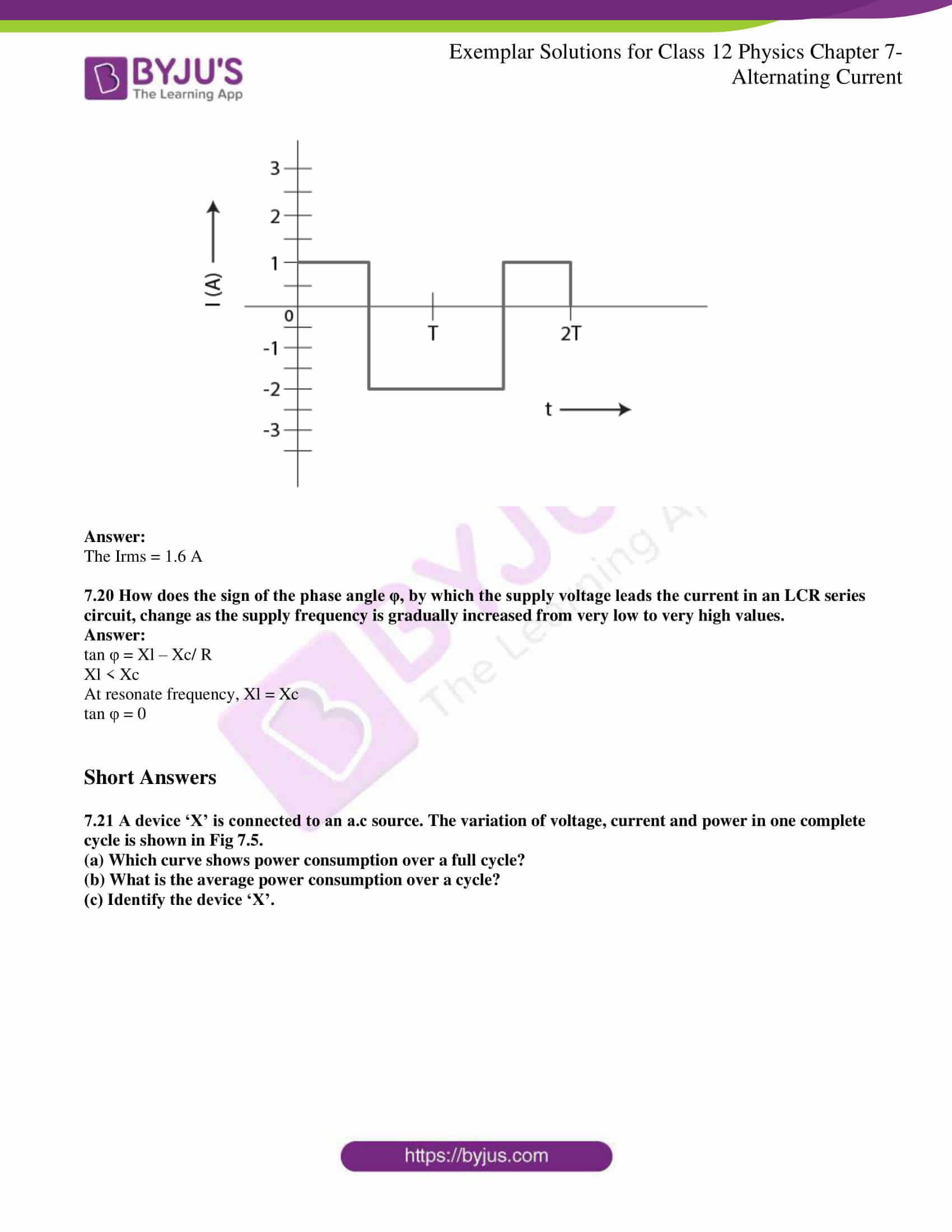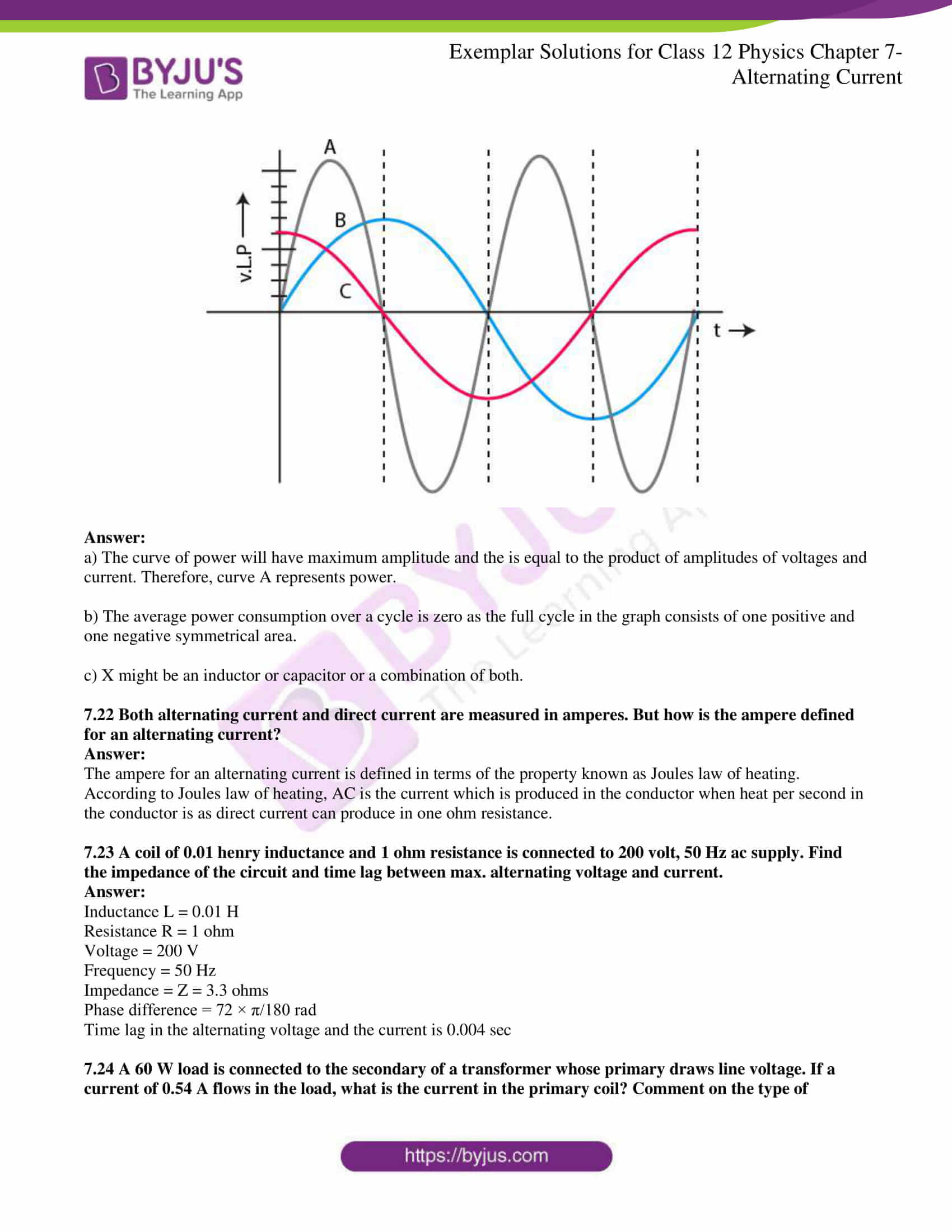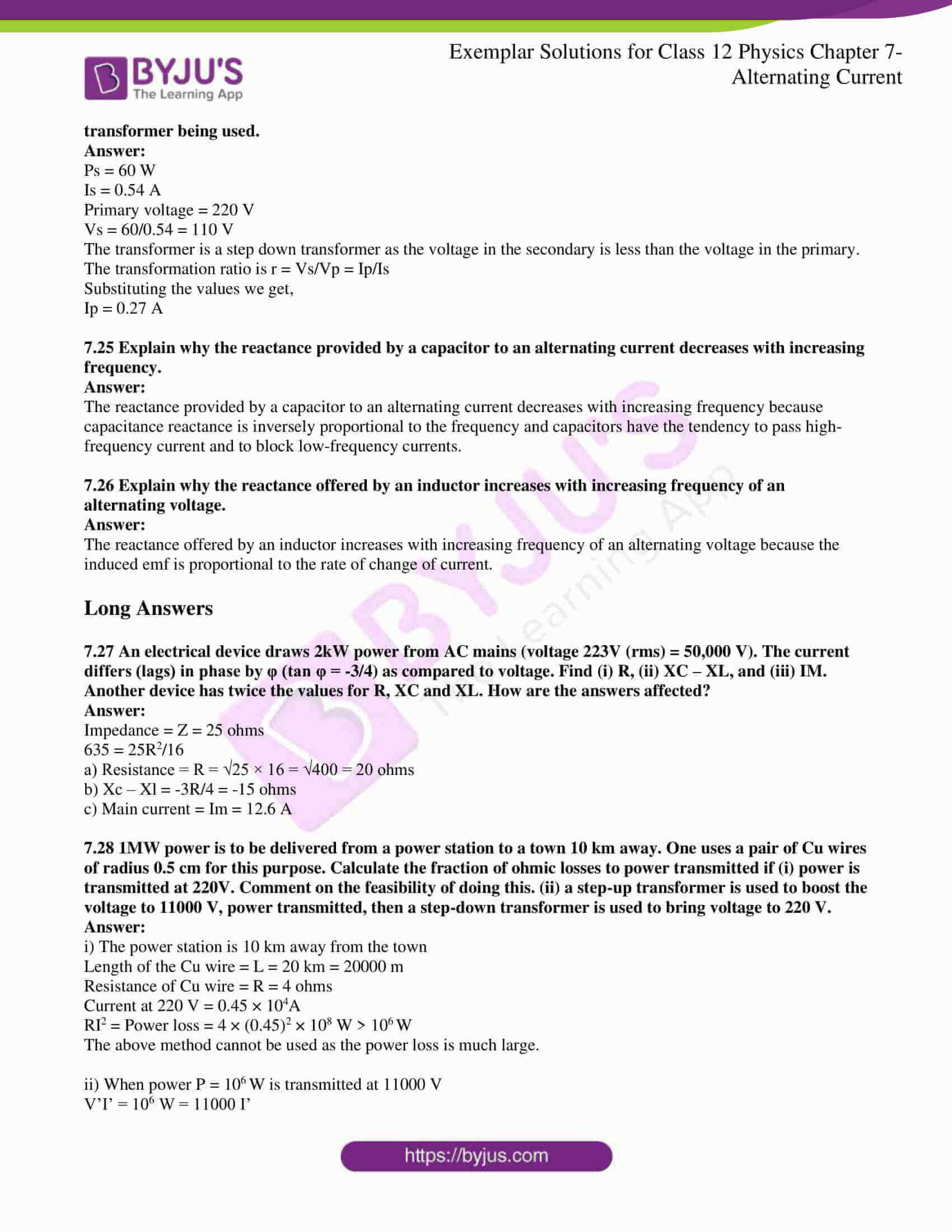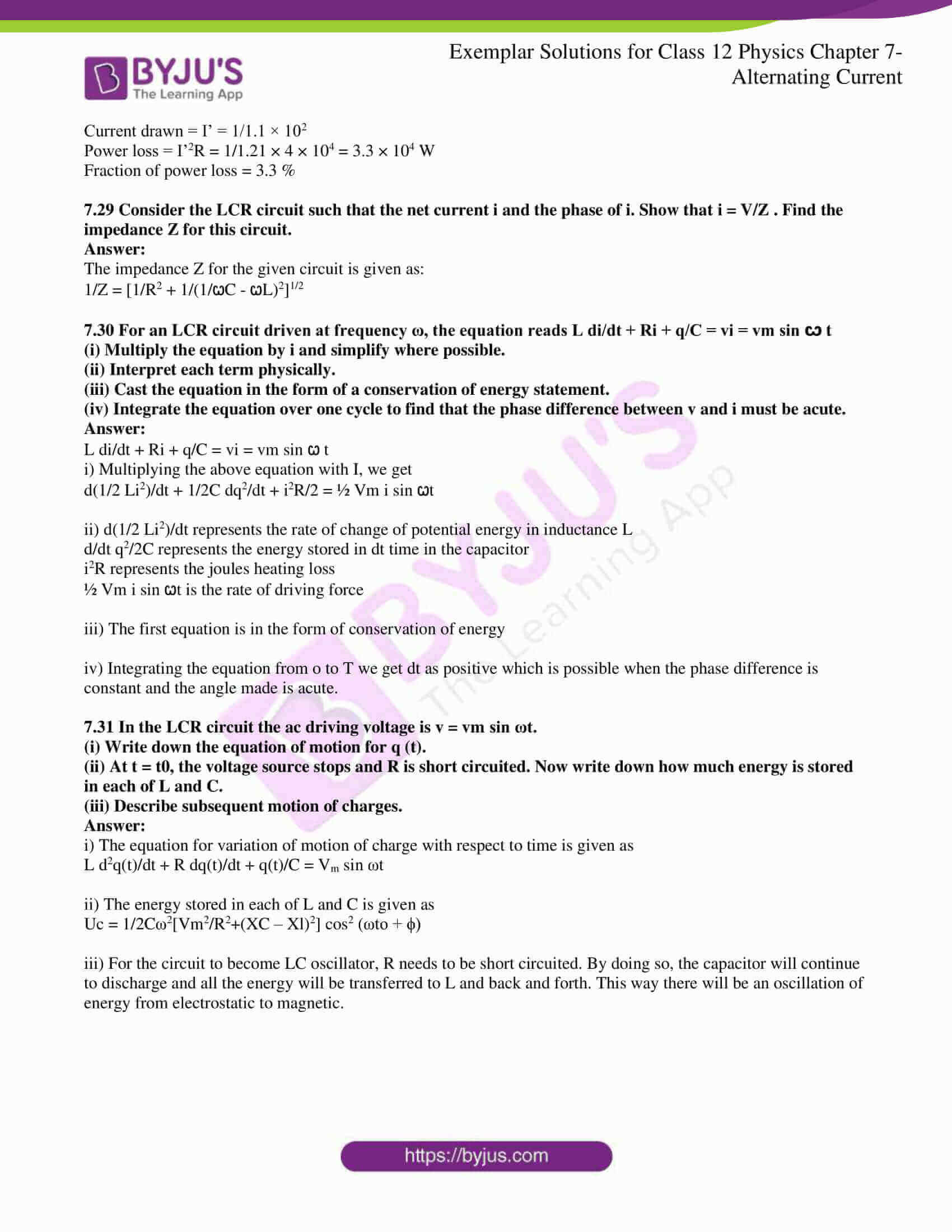### Multiple Choice Questions I

7.1 If the rms current in a 50 Hz ac circuit is 5 A, the value of the current 1/300 seconds after its value becomes zero is

(a) 5 √2 A

(b) 5 √3/2 A

(c) 5/6 A

(d) 5/ √2 A

(b) 5 √3/2 A

7.2 An alternating current generator has an internal resistance Rg and an internal reactance Xg. It is used to supply power to a passive load consisting of a resistance Rg and a reactance XL. For maximum power to be delivered from the generator to the load, the value of XL is equal to

(a) zero.

(b) Xg.

(c) – Xg.

(d) Rg .

(c) – Xg.

7.3 When a voltage measuring device is connected to AC mains, the meter shows the steady input voltage of 220V. This means

(a) input voltage cannot be AC voltage, but a DC voltage.

(b) maximum input voltage is 220V.

(c) the meter reads not v but <v2> and is calibrated to read √<v2> .

(d) the pointer of the meter is stuck by some mechanical defect.

(c) the meter reads not v but <v2> and is calibrated to read √<v2>

7.4 To reduce the resonant frequency in an LCR series circuit with a generator

(a) the generator frequency should be reduced.

(b) another capacitor should be added in parallel to the first.

(c) the iron core of the inductor should be removed.

(d) dielectric in the capacitor should be removed.

(b) another capacitor should be added in parallel to the first

7.5 Which of the following combinations should be selected for better tuning of an LCR circuit used for communication?

(a) R = 20 Ω, L = 1.5 H, C = 35µF.

(b) R = 25 Ω, L = 2.5 H, C = 45µF.

(c) R = 15 Ω, L = 3.5 H, C = 30µF.

(d) R = 25 Ω, L = 1.5 H, C = 45µF.

(c) R = 15 Ω, L = 3.5 H, C = 30µF.

7.6 An inductor of reactance 1 Ω and a resistor of 2 Ω are connected in series to the terminals of a 6 V (rms) a.c. source. The power dissipated in the circuit is

(a) 8 W.

(b) 12 W.

(c) 14.4 W.

(d) 18 W.

(c) 14.4 W.

7.7 The output of a step-down transformer is measured to be 24 V when connected to a 12 watt light bulb. The value of the peak current is

(a) 1/ √2 A.

(b) √2 A.

(c) 2 A.

(d) 2 √2 A

(a) 1/ √2 A.

### Multiple Choice Questions II

7.8 As the frequency of an ac circuit increases, the current first increases and then decreases. What combination of circuit elements is most likely to comprise the circuit?

(a) Inductor and capacitor.

(b) Resistor and inductor.

(c) Resistor and capacitor.

(d) Resistor, inductor and capacitor.

(a) Inductor and capacitor.

(d) Resistor, inductor and capacitor.

7.9 In an alternating current circuit consisting of elements in series, the current increases on increasing the frequency of supply. Which of the following elements are likely to constitute the circuit?

(a) Only resistor.

(b) Resistor and an inductor.

(c) Resistor and a capacitor.

(d) Only a capacitor.

(c) Resistor and a capacitor.

(d) Only a capacitor.

7.10 Electrical energy is transmitted over large distances at high alternating voltages. Which of the following statements is (are) correct?

(a) For a given power level, there is a lower current.

(b) Lower current implies less power loss.

(c) Transmission lines can be made thinner.

(d) It is easy to reduce the voltage at the receiving end using step-down transformers.

(a) For a given power level, there is a lower current.

(b) Lower current implies less power loss.

(d) It is easy to reduce the voltage at the receiving end using step-down transformers.

7.11 For an LCR circuit, the power transferred from the driving source to the driven oscillator is P = I2Z cos φ.

(a) Here, the power factor cos 0, 0. φ ≥ ≥ P

(b) The driving force can give no energy to the oscillator (P = 0) in some cases.

(c) The driving force cannot syphon out (P < 0) the energy out of oscillator.

(d) The driving force can take away energy out of the oscillator.

(a) Here, the power factor cos 0, 0. φ ≥ ≥ P

(b) The driving force can give no energy to the oscillator (P = 0) in some cases.

(c) The driving force cannot syphon out (P < 0) the energy out of oscillator.

7.12 When an AC voltage of 220 V is applied to the capacitor C

(a) the maximum voltage between plates is 220 V.

(b) the current is in phase with the applied voltage.

(c) the charge on the plates is in phase with the applied voltage.

(d) power delivered to the capacitor is zero.

(c) the charge on the plates is in phase with the applied voltage.

(d) power delivered to the capacitor is zero.

7.13 The line that draws power supply to your house from street has

(a) zero average current.

(b) 220 V average voltage.

(c) voltage and current out of phase by 90°.

(d) voltage and current possibly differing in phase φ such that |φ| < π/2.

(a) zero average current.

(d) voltage and current possibly differing in phase φ such that |φ| < π/2.

7.14 If an LC circuit is considered analogous to a harmonically oscillating spring block system, which energy of the LC circuit would be analogous to potential energy and which one analogous to kinetic energy?

The magnetic energy of the LC circuit would be analogous to the kinetic energy while electrostatic energy due to change in charge of a capacitor would be analogous to the potential energy.

7.15 Draw the effective equivalent circuit of the circuit shown in Fig 7.1, at very high frequencies and find the effective impedance.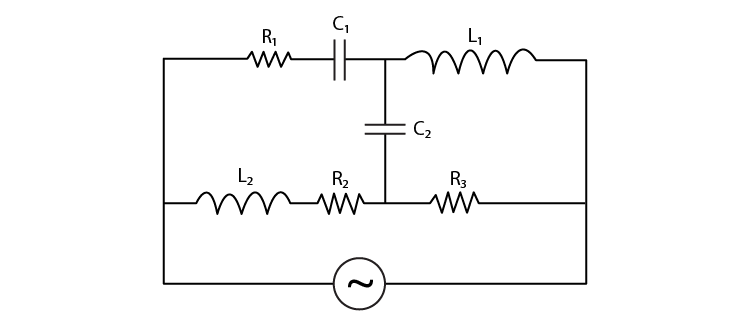The effective impedance = Zeq = R1 + R3

7.16 Study the circuits (a) and (b) shown in Fig 7.2 and answer the following questions.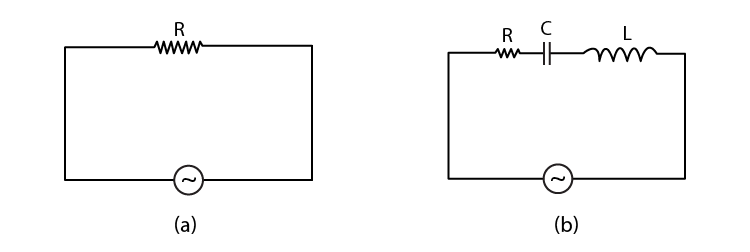(a) Under which conditions would the rms currents in the two circuits be the same? (b) Can the rms current in the circuit (b) be larger than that in (a)?

a) (Irms)a = (Irms)b

Xl = Xc

R = √R2 + (Xl – Xc)2

Therefore, Irms will be equal in (a) as well (b) if Xl = Xc

b) For (Irms)b > (Irms)a

√R2 + (Xl – Xc)2 < R

(Xl < Xc)2 < 0

Reactance cannot be negative.

7.17 Can the instantaneous power output of an ac source ever be negative? Can the average power output be negative?

a) The instantaneous power output of the AC source can be negative when P < 0

b) The average power output cannot be negative as Pav > 0.

7.18 In series LCR circuit, the plot of I max vs ω is shown in Fig 7.3. Find the bandwidth and mark in the figure.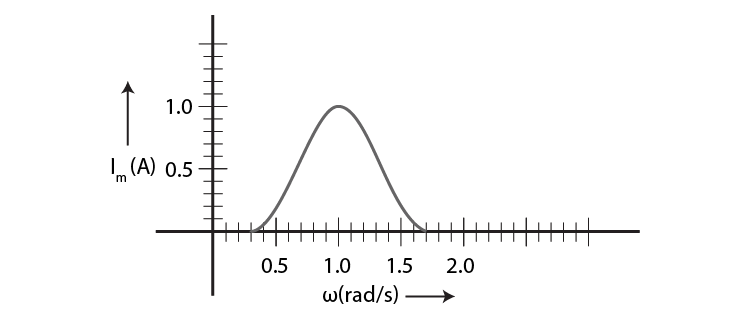7.19 The alternating current in a circuit is described by the graph shown in Fig 7.4. Show rms current in this graph.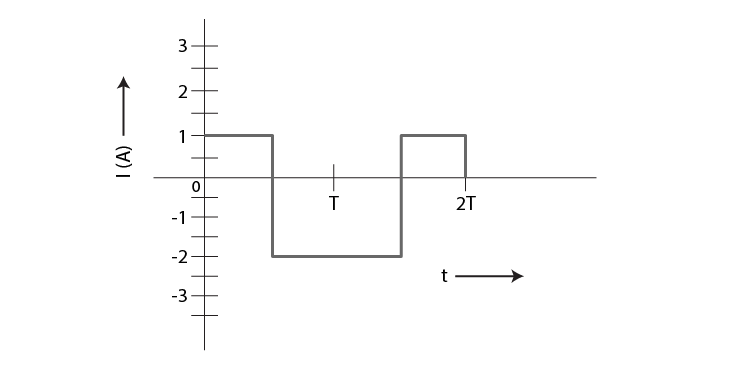The Irms = 1.6 A

7.20 How does the sign of the phase angle φ, by which the supply voltage leads the current in an LCR series circuit, change as the supply frequency is gradually increased from very low to very high values.

tan φ = Xl – Xc/ R

Xl < Xc

At resonate frequency, Xl = Xc

tan φ = 0

7.21 A device ‘X’ is connected to an a.c source. The variation of voltage, current and power in one complete cycle is shown in Fig 7.5.

(a) Which curve shows power consumption over a full cycle?

(b) What is the average power consumption over a cycle?

(c) Identify the device ‘X’.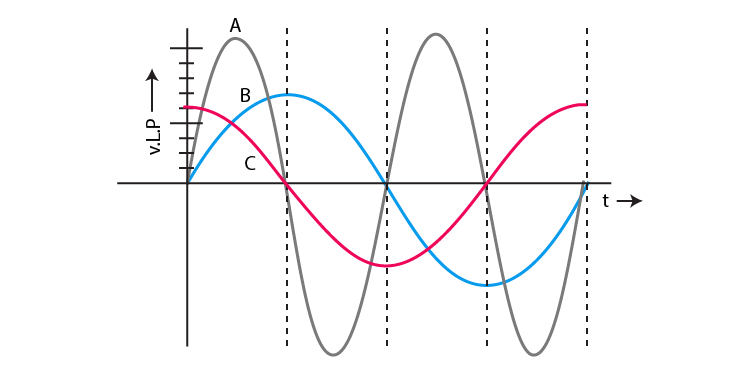a) The curve of power will have maximum amplitude and the is equal to the product of amplitudes of voltages and current. Therefore, curve A represents power.

b) The average power consumption over a cycle is zero as the full cycle in the graph consists of one positive and one negative symmetrical area.

c) X might be an inductor or capacitor or a combination of both.

7.22 Both alternating current and direct current are measured in amperes. But how is the ampere defined for an alternating current?

The ampere for an alternating current is defined in terms of the property known as Joule’s law of heating. According to Joule’s law of heating, AC is the current which is produced in the conductor when heat per second in the conductor is as direct current can produce in one-ohm resistance.

7.23 A coil of 0.01-henry inductance and 1-ohm resistance is connected to 200 volt, 50 Hz ac supply. Find the impedance of the circuit and time lag between max. alternating voltage and current.

Inductance L = 0.01 H

Resistance R = 1 ohm

Voltage = 200 V

Frequency = 50 Hz

Impedance = Z = 3.3 ohms

Phase difference = 72 × π/180 rad

Time lag in the alternating voltage and the current is 0.004 sec

7.24 A 60 W load is connected to the secondary of a transformer whose primary draws line voltage. If a current of 0.54 A flows in the load, what is the current in the primary coil? Comment on the type of transformer being used.

Ps = 60 W

Is = 0.54 A

Primary voltage = 220 V

Vs = 60/0.54 = 110 V

The transformer is a step-down transformer as the voltage in the secondary is less than the voltage in the primary.

The transformation ratio is r = Vs/Vp = Ip/Is

Substituting the values we get,

Ip = 0.27 A

7.25 Explain why the reactance provided by a capacitor to an alternating current decreases with increasing frequency.

The reactance provided by a capacitor to an alternating current decreases with increasing frequency because capacitance reactance is inversely proportional to the frequency and capacitors have the tendency to pass high-frequency current and to block low-frequency currents.

7.26 Explain why the reactance offered by an inductor increases with increasing frequency of an alternating voltage.

The reactance offered by an inductor increases with increasing frequency of an alternating voltage because the induced emf is proportional to the rate of change of current.

7.27 An electrical device draws 2kW power from AC mains (voltage 223V (rms) = 50,000 V). The current differs (lags) in phase by φ (tan φ = -3/4) as compared to voltage. Find (i) R, (ii) XC – XL, and (iii) IM. Another device has twice the values for R, XC and XL. How are the answers affected?

Impedance = Z = 25 ohms

635 = 25R2/16

a) Resistance = R = √25 × 16 = √400 = 20 ohms

b) Xc – Xl = -3R/4 = -15 ohms

c) Main current = Im = 12.6 A

7.28 1MW power is to be delivered from a power station to a town 10 km away. One uses a pair of Cu wires of radius 0.5 cm for this purpose. Calculate the fraction of ohmic losses to power transmitted if (i) power is transmitted at 220V. Comment on the feasibility of doing this. (ii) a step-up transformer is used to boost the voltage to 11000 V, power transmitted, then a step-down transformer is used to bring the voltage to 220 V.

i) The power station is 10 km away from the town

Length of the Cu wire = L = 20 km = 20000 m

Resistance of Cu wire = R = 4 ohms

Current at 220 V = 0.45 × 104A

RI2 = Power loss = 4 × (0.45)2 × 108 W > 106 W

The above method cannot be used as the power loss is much large.

ii) When power P = 106 W is transmitted at 11000 V

V’I’ = 106 W = 11000 I’

Current drawn = I’ = 1/1.1 × 102

Power loss = I’2R = 1/1.21 × 4 × 104 = 3.3 × 104 W

Fraction of power loss = 3.3 %

7.29 Consider the LCR circuit such that the net current i and the phase of i. Show that i = V/Z . Find the impedance Z for this circuit.

The impedance Z for the given circuit is given as:

1/Z = [1/R2 + 1/(1/ꞷC – ꞷL)2]1/2

7.30 For an LCR circuit driven at frequency ω, the equation reads L di/dt + Ri + q/C = vi = vm sin ꞷ t

(i) Multiply the equation by i and simplify where possible.

(ii) Interpret each term physically.

(iii) Cast the equation in the form of a conservation of energy statement.

(iv) Integrate the equation over one cycle to find that the phase difference between v and i must be acute.

L di/dt + Ri + q/C = vi = vm sin ꞷ t

i) Multiplying the above equation with I, we get

d(1/2 Li2)/dt + 1/2C dq2/dt + i2R/2 = ½ Vm i sin ꞷt

ii) d(1/2 Li2)/dt represents the rate of change of potential energy in inductance L

d/dt q2/2C represents the energy stored in dt time in the capacitor

i2R represents the joules heating loss

½ Vm i sin ꞷt is the rate of driving force

iii) The first equation is in the form of conservation of energy

iv) Integrating the equation from o to T we get dt as positive which is possible when the phase difference is constant and the angle made is acute.

7.31 In the LCR circuit the ac driving voltage is v = vm sin ωt.

(i) Write down the equation of motion for q (t).

(ii) At t = t0, the voltage source stops and R is short-circuited. Now write down how much energy is stored in each of L and C.

(iii) Describe subsequent motion of charges.

i) The equation for variation of motion of charge with respect to time is given as

L d2q(t)/dt + R dq(t)/dt + q(t)/C = Vm sin ωt

ii) The energy stored in each of L and C is given as

Uc = 1/2Cω2[Vm2/R2+(XC – Xl)2] cos2 (ωto + ϕ)

iii) For the circuit to become LC oscillator, R needs to be short-circuited. By doing so, the capacitor will continue to discharge and all the energy will be transferred to L and back and forth. This way there will be an oscillation of energy from electrostatic to magnetic.

An alternating current is a type of electric current which flows in both directions, unlike a direct current, which flows in a single direction. Alternating current is usually represented as a sine waveform and sometimes as triangular waves and square waves. The AC carrier signal is useful for carrying different media, such as sound and video, with the help of modulation.

#### Some of the Applications of Alternating Current

1. Televisions
2. Battery cells